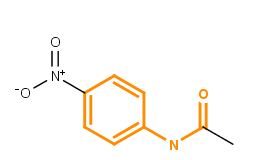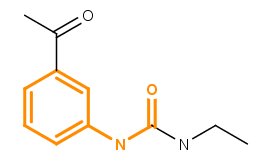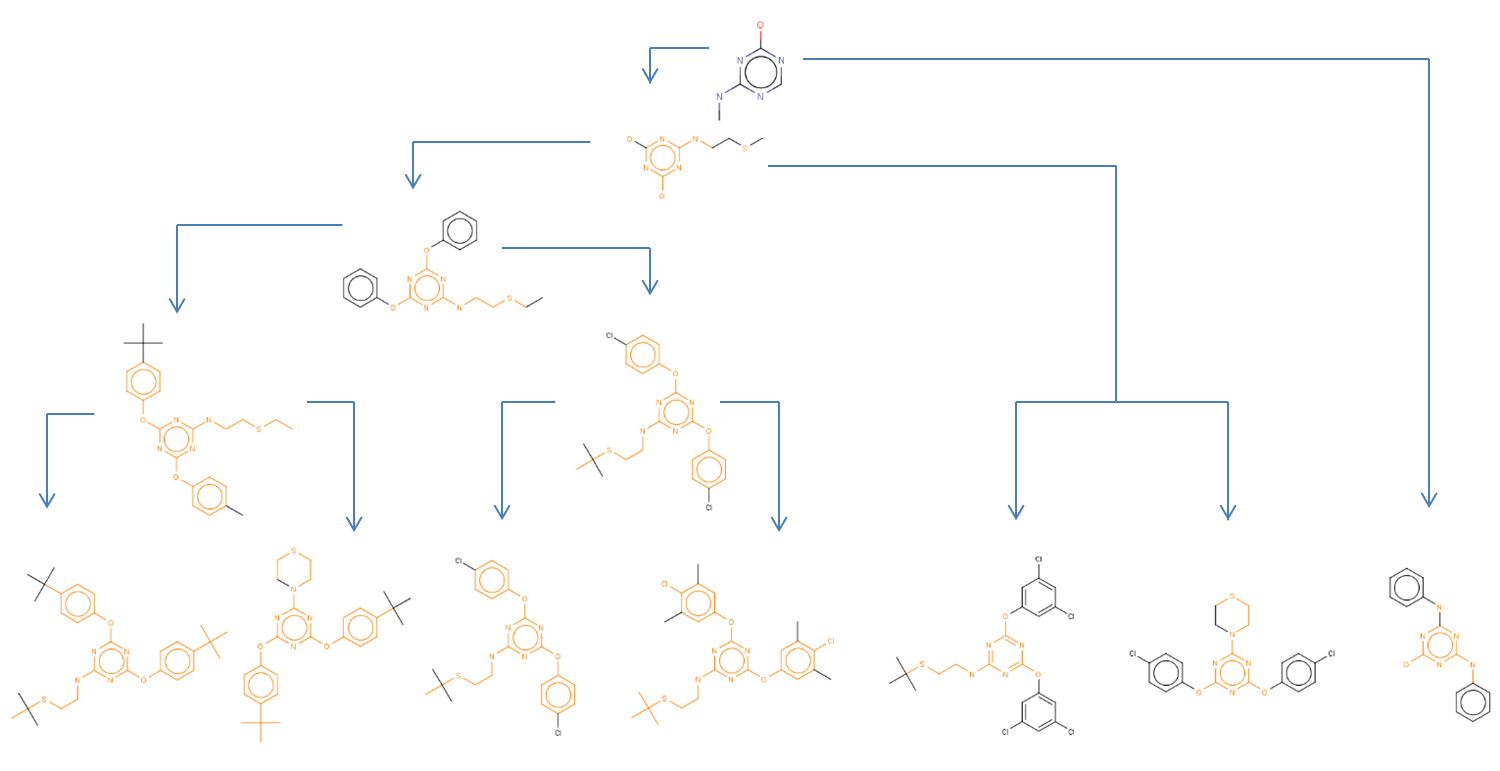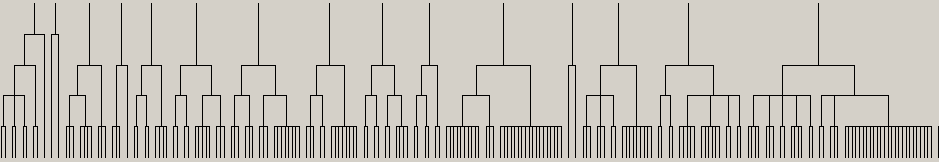# Library MCS (LibMCS) clustering

This manual gives you a walk-through on how to use the Library MCS (LibMCS) clustering:

# Introduction and concept

Library MCS searches for the Maximum Common Substructures (or MCS) of a compound library in a hierarchical manner. The concept of maximum common substructure is defined for two graphs (in this case for two chemical structures) and it refers to the largest subgraph (substructure) common in both structures. For more information about MCS search, see here. The figure below illustrates the maximum common substructure of two compounds; the MCS is highlighted in orange.Fig. 1 Maximum Common Substructure (MCS) of two molecules

The concept of pairwise MCS is not directly applicable for a set of compounds. Two problems arise:

(1) The pairwise comparison of each and every compound (exact method) in a set of compounds is practically infeasible, since it would involve n · ( n - 1 ) / 2 MCS calculations, where n denotes the number of compounds in the library. In the case of 1000 structures 499,500 pairwise calculations are needed. Even one such comparison is fairly complex and requires significant computation time. If, let's say the computation time of one MCS search is 10ms on a certain hardware, the full evaluation of a 1000 library would take 4995 seconds, that is, nearly 1.5 hours (and 35 hours for 5000 molecules).

(2) Unless the set contains structural analogs, that is structures with similar molecular backbone and variations only in their functional groups, the MCS of the analyzed set degenerates easily to a carbon atom. Thus, outliers require careful handling.

The structure-based hierarchical clustering applied by LibMCS offers a feasible approach to overcome the above mentioned problems with two substantial benefits (fast, turbo methods): (1) the number of MCS calculations needed is proportional to n (practically less than n/2) and (2) outliers form singletons thus they do not deteriorate maximal common substructures.

Initial structures are found at the bottom of the hierarchy. The next level contains the MCSs of clusters of initial molecules: all molecules that share a common structure are placed in a cluster (group). This MCS based classification of molecules creates disjoint subsets (clusters), that is, one molecule belongs to one cluster only.
It is not guaranteed, though, that this disjoint classification is optimal. Molecule a can have an MCS ma,b when compared to molecule b, while the comparison against molecule c may result in a different MCS ma,c. Since only one of these two possible comparisons are made in hierarchical MCS clustering, the algorithm may find MCS ma,b even if MCS ma,c is larger. Fortunately, such anomalies occur very rarely, and it is neglectable in practice. The price to be paid for an exact (optimal) solution to be found is high: all possible pairwise comparisons have to be made, which, as seen above is, is costly. A significantly faster solution, however approximate with ignorable error, was preferred. The libmcs application (and the corresponding LibraryMCS java class) is an approach to reach this goal.Fig. 2 Substructure hierarchy

The figure above illustrates the substructure hierarchy as represented by a dendogram of the LibMCS GUI. The hierarchy of the example structures have five levels. The lowest row contains the leaves, that is the structures in the library. The top row contains the smallest common substructure containing at least 9 atoms (as defined by the default settings).Fig. 3 Substructure hierarchy represented by the LibMCS dendogram

# Usage

JChem provides two application modes to run Library MCS: Graphical User Interface and command line interface.

## Command line usage

Many operation of LibMCS can also be invoked without graphical user interface. The libmcs program is the command line interface of the JChem LibMCS application.

The list of options as listed with libmcs -h:

`libmcs [input file] [options] `
`Options:  -h, --help                   this help message  -v, --verbose                progress monitoring and other messages  -e, --exact                  exact MCS recognition  -f, --fast                   fast, yet fairly accurate MCS recognition  -t, --turbo                  fastest and less reliable MCS recognition  -n, --minMCS <size>        integer value specifying the MCS size                               where clustering terminates  -m, --match (a|b|c|r) (+|-)  turns matching constraints on (+), off (-)                               for atom types (a), bond types (b),                               formal charges (c) and rings (r)  -o, --output       SDF output file, terminal if -o omitted  -o, --output CSV   CSV output file  -r, --report                 generate report (cluster statistics)`

### Examples

• Execution of LibMCS on SC100.sdf, and writing output into output.mrv file:

`\$ libmcs examples/molecules/SC100.sdf -o output.mrv `
• Execution of LibMCS on SC100.sdf when atom types are, bond types are not taken into account. The output is displayed on the console:

`\$ libmcs examples/molecules/SC100.sdf -m a+ b- `

## LibMCS Graphical User Interface

Clustering results can be visualized with the graphical user interface of the LibMCS. You can find the detailed description of it here.